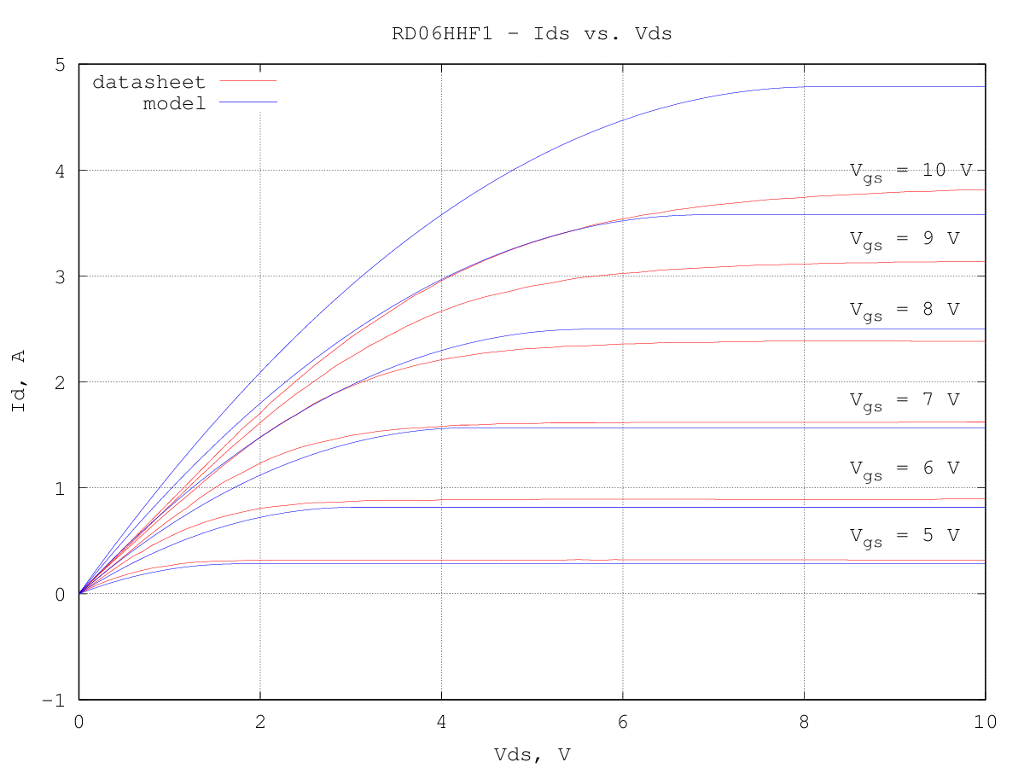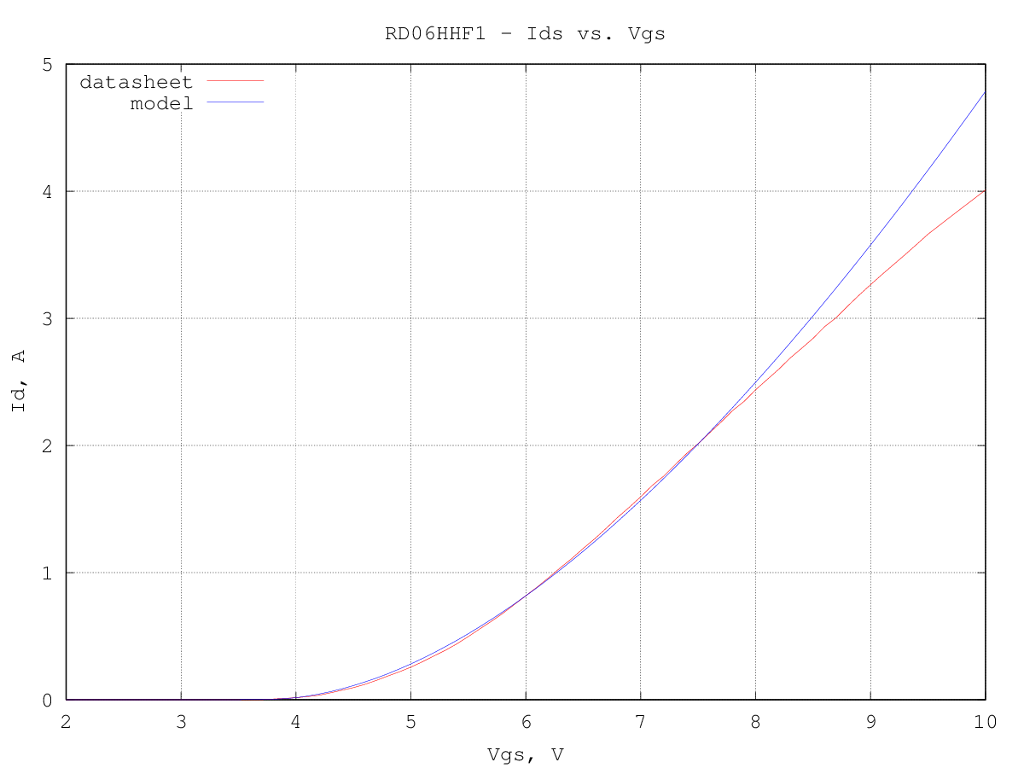# Mitsubishi RD06HHF1 LDMOS LTspice model

Under construction...

The device has been modeled using the LTspice VDMOS model, since it is well suited also for LDMOS devices and contains only few of parameters which can be guessed from the scarce data available from the datasheet.
The static (drain current) and dynamic (capacitances) characteristics were separately optimized, starting from a first guess extracted from the curves in the datasheet. The optimized model description is:

.model RD06HHF1 VDMOS(Rg=1.88m Rd=0m Rs=263m Vto=3.70 Kp=0.377 Lambda=0 mtriode=0.733 subthres=2.18m Cgdmax=5.64p Cgdmin=0.865p Cgs=23.5p Cjo=39.3p M=0.255 Vj=0.372)

the quality of the fit is not particularly good over the entire drain currents range (the VDMOS model is targeted mainly at switching circuits applications and does not have many parameters to optimize the entire range) but is probably accurate enough for the drain current levels usually encountered for this device in amateur radio applications.
Another problem found during the VDMOS model extraction is that even the datasheet graphs are not entirely consistent, as the Ids vs. Vds curves do not exactly agree with the Ids vs. Vgs curves, so the model extracted tries to be a best compromise between the two curves...

Here below is a graph of the drain current vs. the drain voltages for several values of the gate voltage; as said, the fit is relatively good at low/medium drain currents and not very good in the linear region:The Ids vs. Vgs curve fit, for a fixed drain voltage of 10 V, is good up to a couple of amps of drain current:Input (Ciss) and output (Coss) capacitances are well modeled, while the reverse transfer capacitance (Crss) is quite off, as the voltage dependency is not matching with the one implemented in the LTspice model: